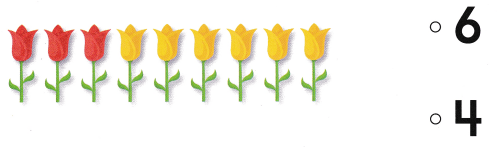# Texas Go Math Kindergarten Lesson 14.3 Answer Key Differences Within 10

Refer to our Texas Go Math Kindergarten Answer Key Pdf to score good marks in the exams. Test yourself by practicing the problems from Texas Go Math Kindergarten Lesson 14.3 Answer Key Differences Within 10.

## Texas Go Math Kindergarten Lesson 14.3 Answer Key Differences Within 10

Essential Question
How can you show differences within 10?

Explore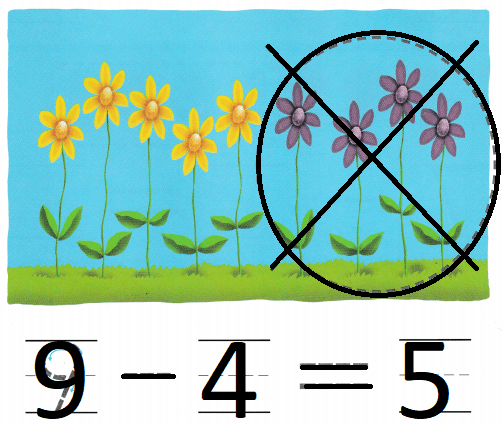Explanation:
There are 9 flowers
4 flowers are purple
9 – 4 = 5
so, 5 flowers are yellow.

Directions
There are nine flowers. Four flowers are purple. Trace the circle and X around those flowers, The rest of the flowers are yellow. How many flowers are yellow? Trace and write to complete the subtraction sentence.

Share and Show

Question 1.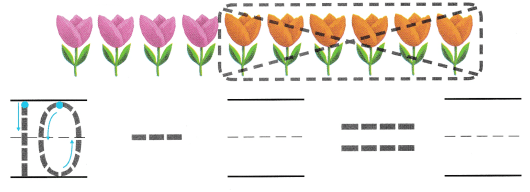Explanation:
There are 10 flowers
6 tulips are orange
10 – 6 = 4
so, 4 tulips are pink.

Question 2.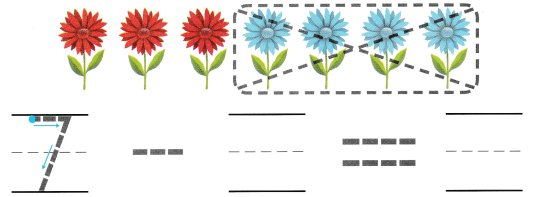Explanation:
There are 7 flowers
4 flowers are blue
7 – 4 = 3
so, 3 flowers are red.

Question 3.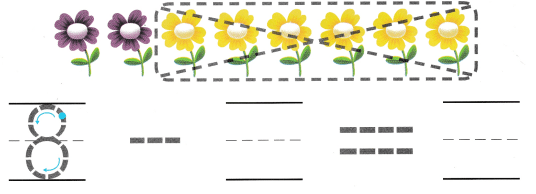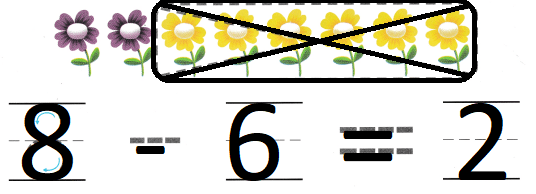Explanation:
There are 8 flowers
6 are yellow flowers
8 – 6 = 2
so, 2 flowers are purple.

Directions
1. Listen to the subtraction word problem. There are ten flowers. Six of the flowers are orange. The rest are pink. Trace the circle and X, How many flowers are pink? Trace and write to complete the subtraction sentence. 2-3. Listen to the subtraction word problem. Trace and write to complete the subtraction sentence.

Question 4.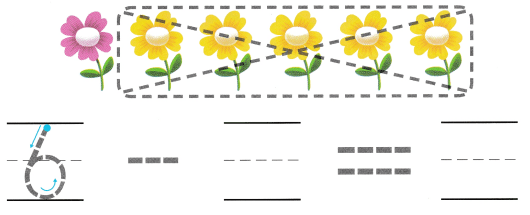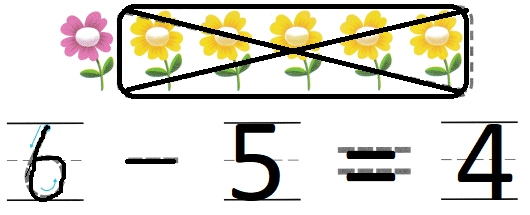Explanation:
There are 6 flowers
5 flowers are yellow
6 – 5 = 1
so, 1 flower is pink.

Question 5.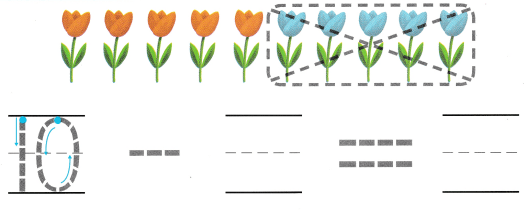Explanation:
There are 10 tulips
5 tulips are blue
10 – 5 = 5
so, 5 tulips are orange.

Question 6.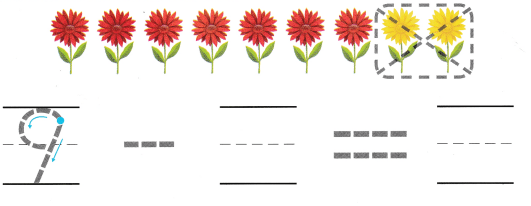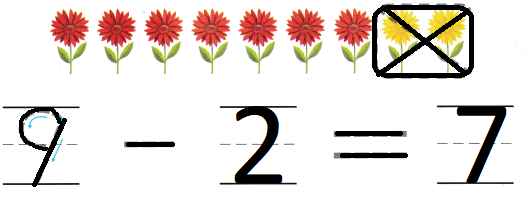Explanation:
There are 9 flowers
2 are yellow flowers
9 – 2 = 7
so, 7 flowers are red.

Directions
4-6. Listen to the subtraction word problem. Trace and write to complete the subtraction sentence.

Problem Solving

Question 7.Explanation:
There are 10 fruits
one is eaten
10 – 1 = 9
so, 9 fruits are left.

Question 8.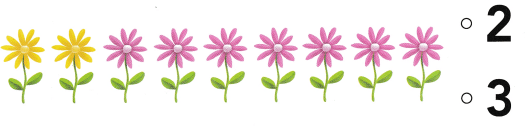Explanation:
There are nine flowers.
Seven flowers are pink.
The rest are yellow.
9 – 7 = 2
so, 2 are yellow flowers.

Directions
7. Complete the subtraction sentence. Draw to show what you know about this subtraction sentence. Tell a friend about your drawing. 8. Choose the correct answer There are nine flowers. Seven flowers are pink. The rest are yellow. Who? is 9 – 7?

### Texas Go Math Kindergarten Lesson 14.3 Homework and Practice

Question 1.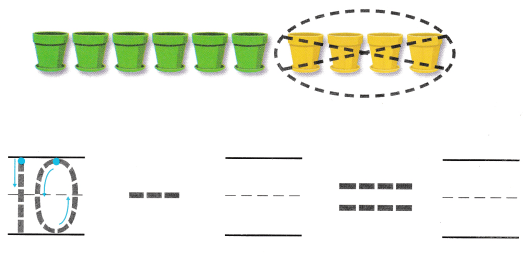Explanation:
There are ten flowerpots.
4 flower pots are yellow
10 – 4 = 6
so, 6 flowerpots are green

Question 2.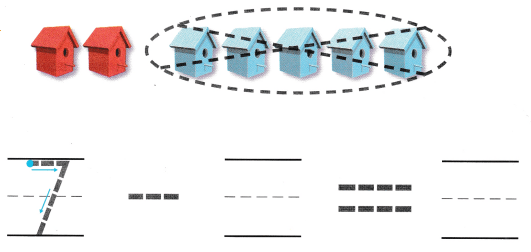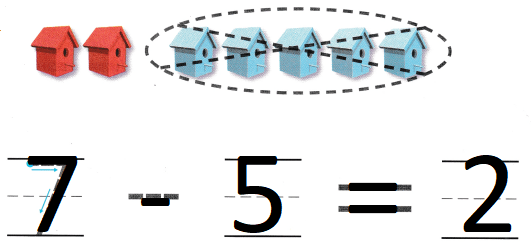Explanation:
There are seven birdhouses.
5 are blue
7 – 5 = 2
2 birdhouses are red

Directions
Trace and write to complete the subtraction sentence 1. There are ten flowerpots. How many flowerpots are green? 2. There are seven birdhouses. How many birdhouses are red?

Lesson Check

Question 3.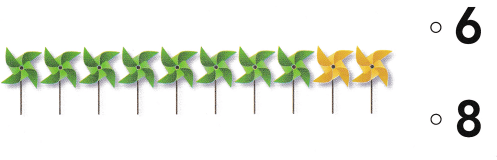Explanation:
There are 10 pinwheels.
Two of them are yellow.
10 – 2 = 8
so, 8 are green pin wheels.

Question 4.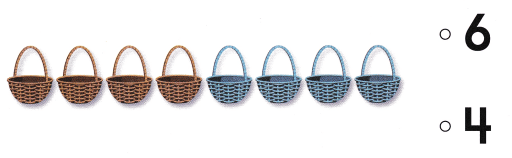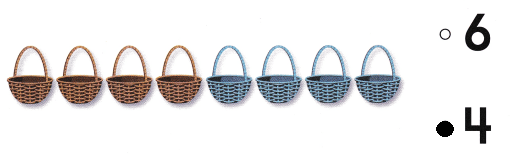Explanation:
Four of them are blue.
8 – 4 = 4

Question 5.